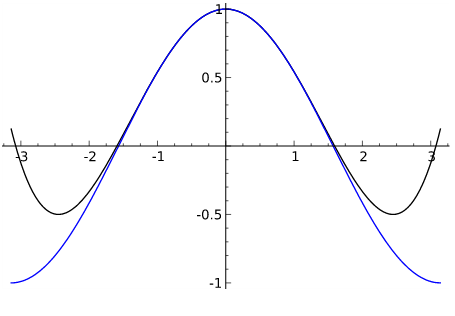# Neither Odd Nor Even?

Algebra Level 3How many function(s) with domain $\mathbb{R}$ and range $\subseteq \mathbb{R}$ are both even and odd?

×